# User:IssaRice/Computability and logic/S–m–n theorem

The smn theorem (also called the parametrization theorem) states that if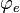$\varphi_e$ is an$(m+n)$ place computable partial function and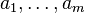$a_1,\ldots,a_m$ are natural numbers, then there exists a primitive recursive function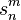$s^m_n$ such that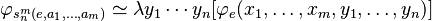$\varphi_{s^m_n(e,a_1,\ldots,a_m)} \simeq \lambda y_1 \cdots y_n [\varphi_e(x_1,\ldots,x_m,y_1,\ldots,y_n)]$.

In other words, the theorem states that if we start out with a computable partial function$\varphi_e$ of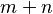$m+n$ variables, then we can fill in$m$ of the variables with actual values. When we do this, the resulting$n$-place partial function continues to be computable. Moreover, we can find the index of this new partial function in terms of the old index and the values in a primitive recursive way.

To give an example, suppose we start out with the function$\varphi_e = \lambda x,y . x+y$. Then we can "fill in" one of the variables with a value, e.g. by taking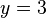$y=3$. Now we have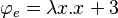$\varphi_e = \lambda x.x+3$. The smn theorem claims that this resulting function is also computable. It also says that we can find the source code of this new function using$e$ (the original source code) and 3 (the value we are substituting).

In terms of programming, the smn theorem says that if we start out with a computer program taking several arguments, then we can transform the source code of the program into another computer program that does the same thing except with some of the variables filled in. e.g. if we have def f(x, y): return x + y and we want y=3, we can easily transform this into def g(x): y=3; return x + y. To perform this translation, all we needed was the original source code (for f) as well as the value we are substituting (y=3). The smn theorem claims that (1) the result is computable (is a computer program); and (2) the transformation is primitive recursive (can be computed using only bounded loops).

The smn theorem is essentially the same thing as currying in functional programming languages.

The smn theorem, together with the universal function, is useful for proving other theorems and also to avoid the arbitrariness of the specific encoding used.

Different kinds of proofs:

• Tape/register-based way
• Using recursive functions and ways of encoding them

## Etymology

The name "smn" comes from the notation$s^m_n$ (denoted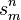$s^n_m$ in some texts) for the function that finds the new index.